# NCERT Solutions for Class 8 Maths Chapter 9 Exercise 9.1 – Algebraic Expressions & Identities

NCERT Solutions for Class 8 Maths Chapter 9 Exercise 9.1 – Algebraic Expressions & Identities, has been designed by the NCERT to test the knowledge of the student on the following topics:-

• What are Expressions?
• Terms, Factors and Coefficients
• Monomials, Binomials and Polynomials
• Like and Unlike Terms
• Addition and Subtraction of Algebraic Expressions

### NCERT Solutions for Class 8 Maths Chapter 9 Exercise 9.1 – Algebraic Expressions & Identities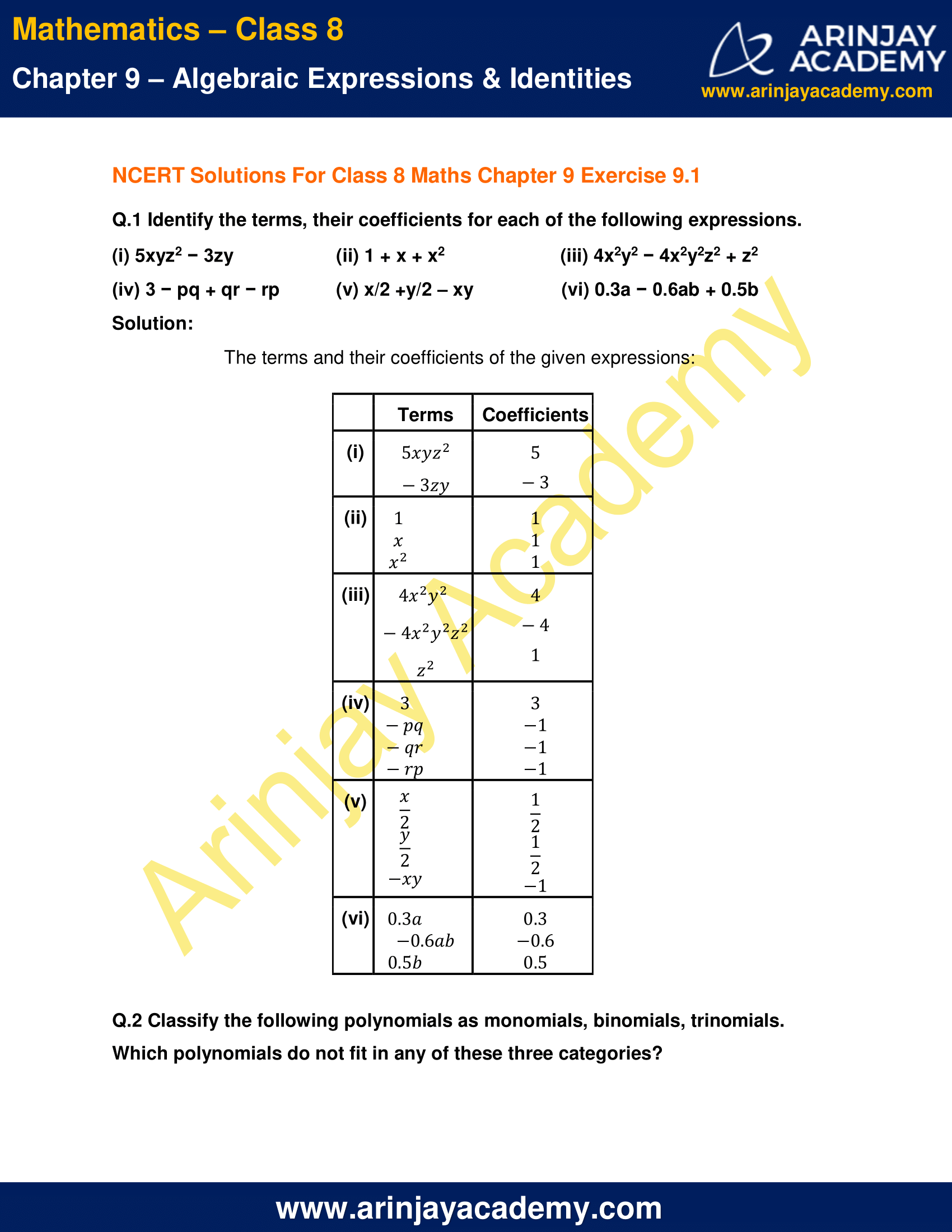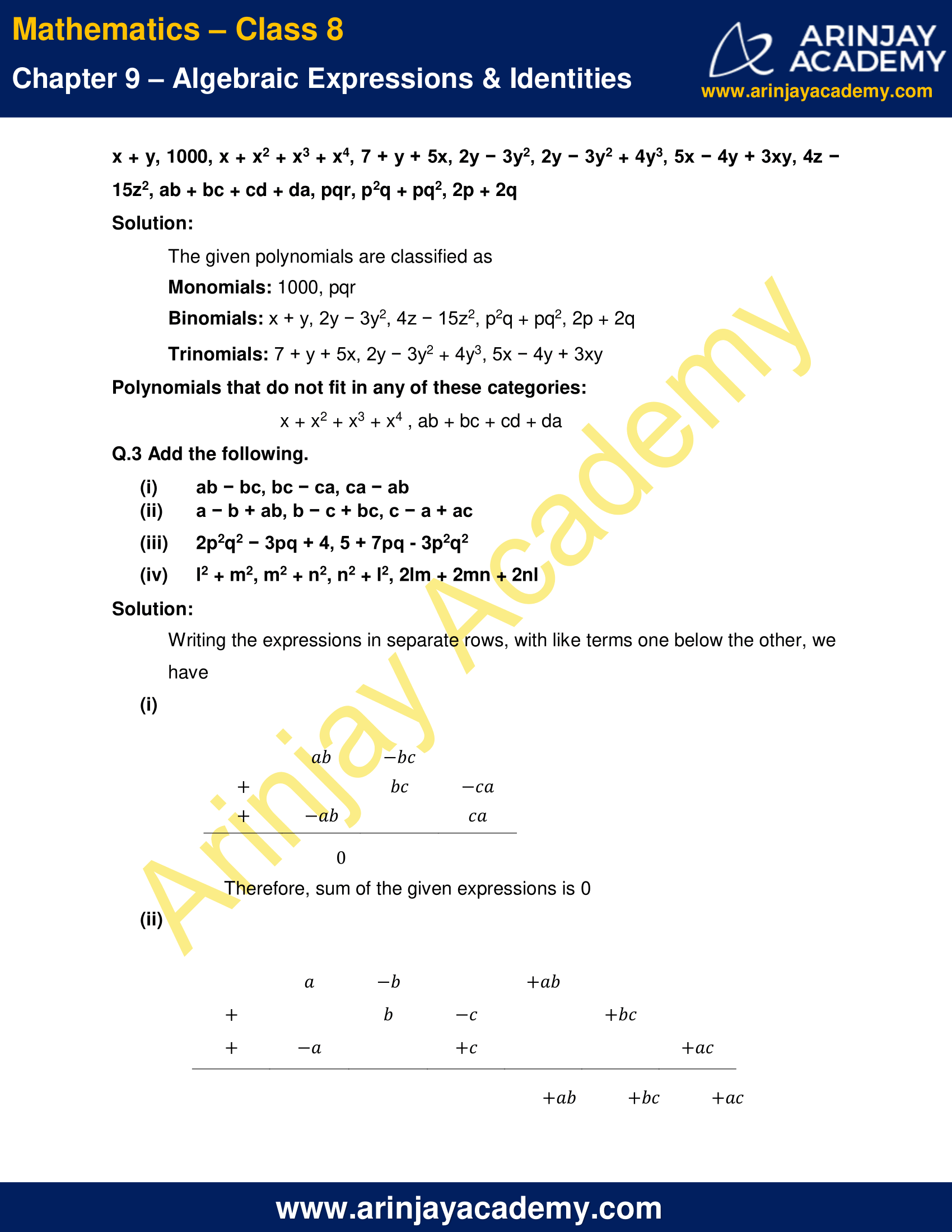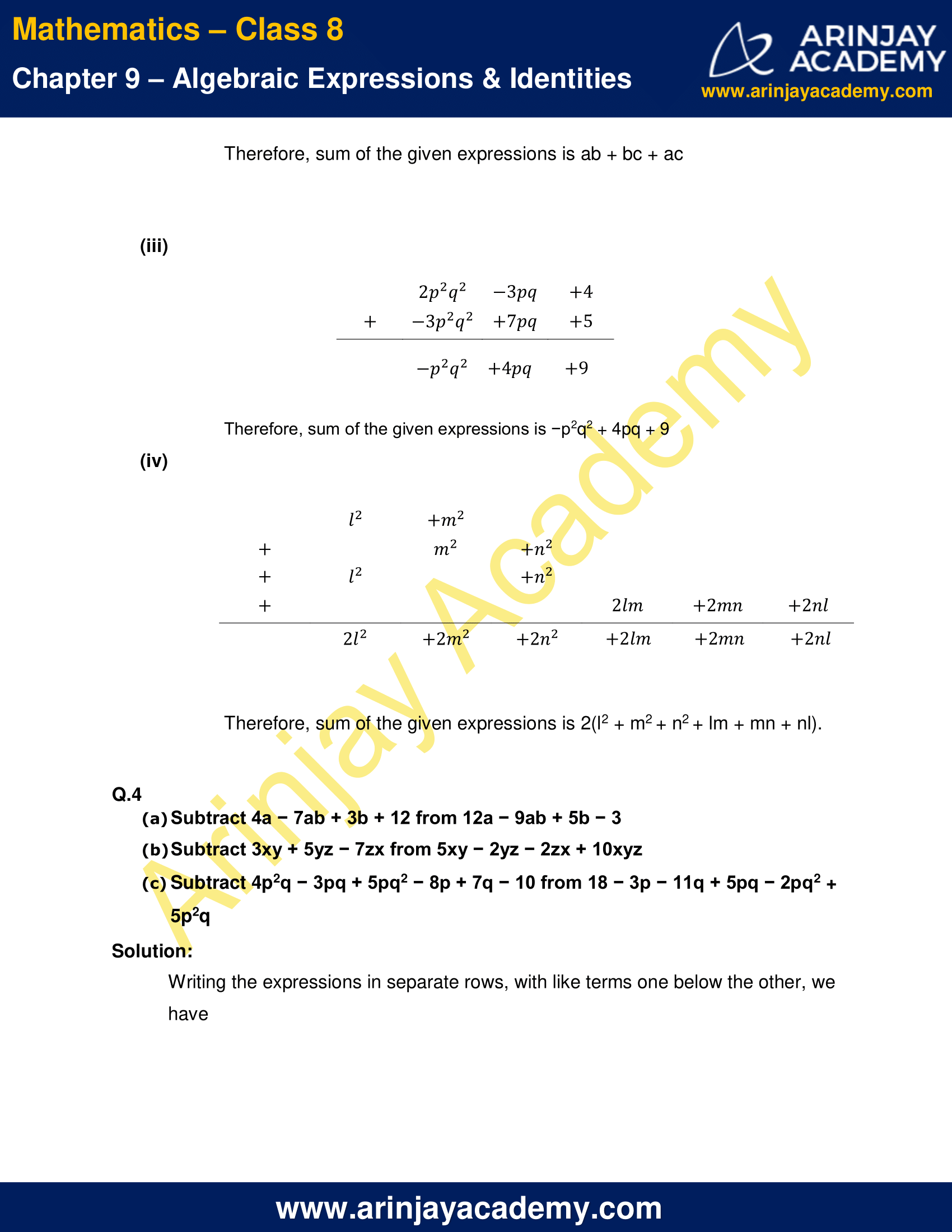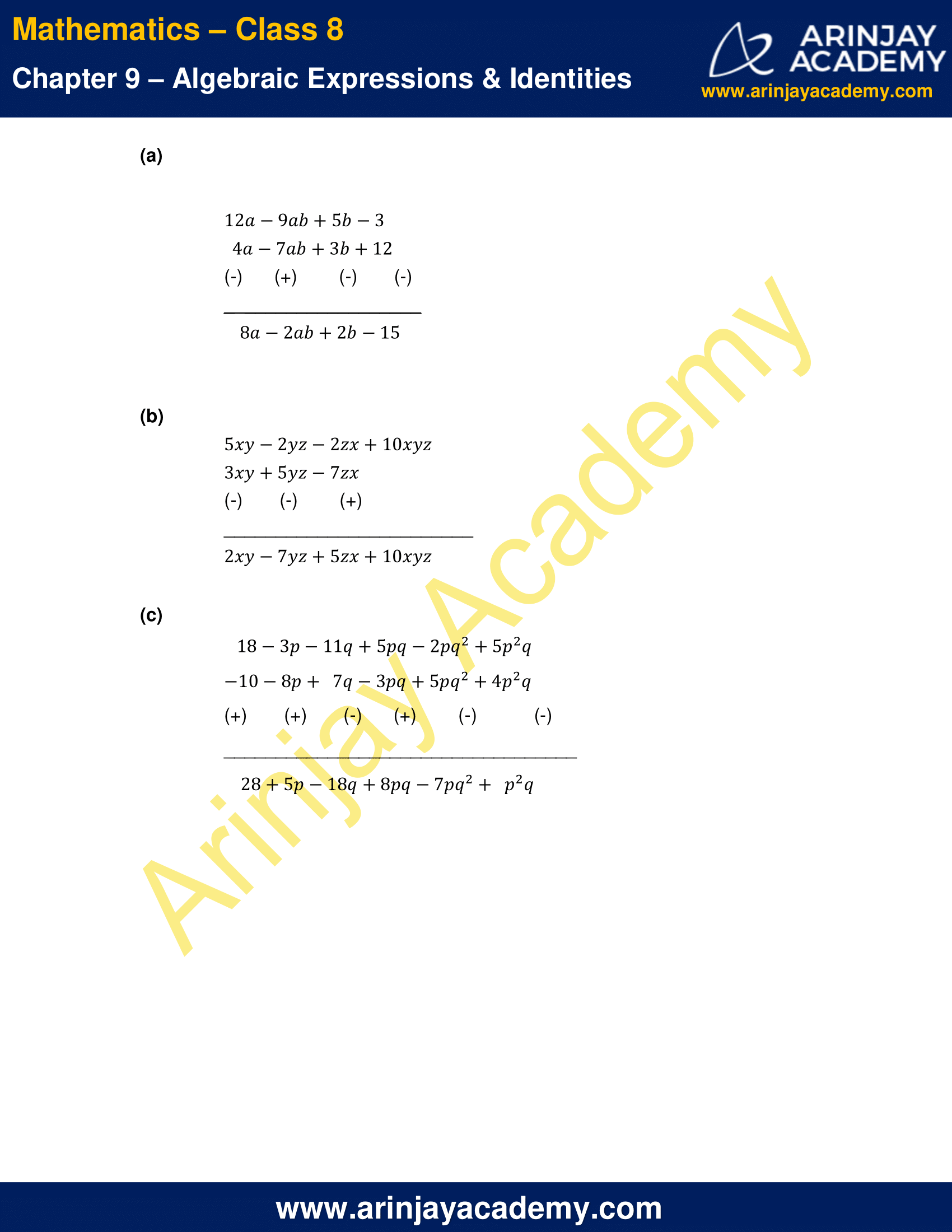NCERT Solutions for Class 8 Maths Chapter 9 Exercise 9.1 – Algebraic Expressions & Identities

Q.1 Identify the terms, their coefficients for each of the following expressions.

(i) 5xyz2 − 3zy
(ii) 1 +
x + x2
(iii) 4
x2y2 − 4x2y2z2 + z2
(iv) 3 − pq + qrrp
(v) x/2 +y/2 – xy
(vi) 0.3
a − 0.6ab + 0.5b

Solution:

The terms and their coefficients of the given expressions: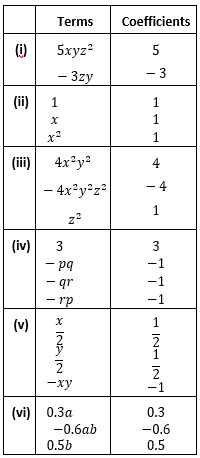Q.2 Classify the following polynomials as monomials, binomials, trinomials. Which polynomials do not fit in any of these three categories?

x + y, 1000, x + x2 + x3 + x4, 7 + y + 5x, 2y − 3y2, 2y − 3y2 + 4y3, 5x − 4y + 3xy, 4z − 15z2, ab + bc + cd + da, pqr, p2q + pq2, 2p + 2q

Solution:

The given polynomials are classified as

Monomials: 1000, pqr
Binomials: x + y, 2y − 3y2, 4z − 15z2, p2q + pq2, 2p + 2q
Trinomials: 7 + y + 5x, 2y − 3y2 + 4y3, 5x − 4y + 3xy
Polynomials that do not fit in any of these categories: x + x2 + x3 + x4 , ab + bc + cd + da

(i) ab − bc, bc − ca, ca − ab
(ii) a − b + ab, b − c + bc, c − a + ac
(iii) 2p2q2 − 3pq + 4, 5 + 7pq – 3p2q2
(iv) l2 + m2, m2 + n2, n2 + l2, 2lm + 2mn + 2nl

Solution:

Writing the expressions in separate rows, with like terms one below the other, we have

(i)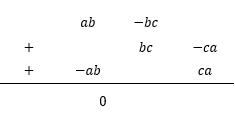Therefore, sum of the given expressions is 0

(ii)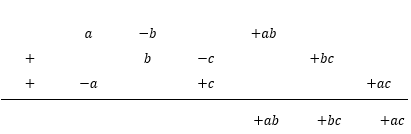Therefore, sum of the given expressions is ab + bc + ac

(iii)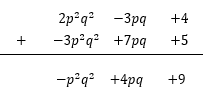Therefore, sum of the given expressions is −p2q2 + 4pq + 9

(iv)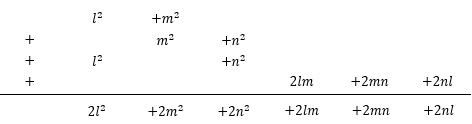Therefore, sum of the given expressions is 2(l2 + m2 + n2 + lm + mn + nl).

Q.4

(a) Subtract 4a − 7ab + 3b + 12 from 12a − 9ab + 5b − 3

(b) Subtract 3xy + 5yz − 7zx from 5xy − 2yz − 2zx + 10xyz

(c) Subtract 4p2q − 3pq + 5pq2 − 8p + 7q − 10 from 18 − 3p − 11q + 5pq − 2pq2 + 5p2q

Solution:

Writing the expressions in separate rows, with like terms one below the other, we have

(a)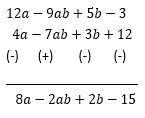(b)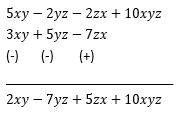(c)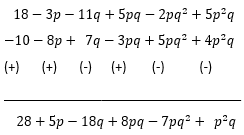The next Exercise for NCERT Solutions for Class 8 Maths Chapter 9 Exercise 9.2 – Algebraic Expressions and Identities can be accessed by clicking here

NCERT Solutions For Class 8 Maths Chapter 9 Exercise 9.1 – Algebraic Expressions & Identities